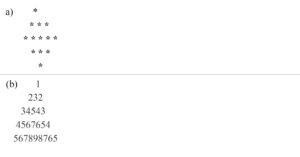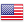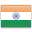Grade "A+" Accredited by NAAC with a CGPA of 3.46
Grade "A+" Accredited by NAAC with a CGPA of 3.46

# Programming in Python

Course ID
BHCS 19B
Level
Program
B.Sc. CS (Hons.)
Semester
Third
Credits
4.0
Paper Type
Skill Enhancement - 1
Method
Lecture & Practical

## Unique Paper Code: Update Awaited

This course is designed to introduce the student to the basics of programming using Python. The course covers the topics essential for developing well documented modular programs using different instructions and built-in data structures available in Python.

## Learning Outcomes:

At the end of the course, students should be able to:

• Develop, document, and debug modular python programs to solve computational problems.
• Select a suitable programming construct and data structure for a situation.
• Use built-in strings, lists, sets, tuples and dictionary in applications.
• Define classes and use them in applications.
• Use files for I/O operations.

## Course Contents

Unit 1
Unit 2
Unit 3
Unit 4
Unit 5
Unit 6

Unit 1

Introduction to Programming using Python: Structure of a Python Program, Functions, Interpreter shell, Indentation. Identifiers and keywords, Literals, Strings, Basic operators (Arithmetic operator, Relational operator, Logical or Boolean operator, Assignment Operator, Bit wise operator).

Unit 2

Building blocks of Python: Standard libraries in Python, notion of class, object and method.

Unit 3

Creating Python Programs: Input and Output Statements, Control statements:-branching, looping, Exit function, break, continue and pass, mutable and immutable structures. Testing and debugging a program.

Unit 4

Built-in data structures: Strings, lists, Sets, Tuples and Dictionary and associated operations. Basic searching and sorting methods using iteration and recursion.

Unit 5

Visualization using 2D and 3D graphics: Visualization using graphical objects like Point, Line, Histogram, Sine and Cosine Curve, 3D objects.

Unit 6

Exception Handling and File Handling: Reading and writing text and structured files, Errors and Exceptions.

### Practicals

#### Lab List 1

1. Execution of expressions involving arithmetic, relational, logical, and bitwise operators in the shell window of Python IDLE.
2. Write a Python function to produce the outputs such as:1. Write a Python program to illustrate the various functions of the “Math” module.
2. Write a function that takes the lengths of three sides: side1, side2 and side3 of the triangle as the input from the user using input function and return the area of the triangle as the output. Also, assert that sum of the length of any two sides is greater than the third side.
3. Consider a showroom of electronic products, where there are various salesmen. Each salesman is given a commission of 5%, depending on the sales made per month. In case the sale done is less than 50000, then the salesman is not given any commission. Write a function to calculate total sales of a salesman in a month, commission and remarks for the salesman. Sales done by each salesman per week is to be provided as input. Assign remarks according to the following criteria:
Excellent: Sales >=80000
Good: Sales>=60000 and <80000
Average: Sales>=40000 and <60000
Work Hard: Sales < 40000
4. Write a Python function that takes a number as an input from the user and computes its factorial.
5. Write a Python function to return nth terms of Fibonacci sequence.
6. Write a function that takes a number with two or more digits as an input and finds its reverse and computes the sum of its digits.
7. Write a function that takes two numbers as input parameters and returns their least common multiple and highest common factor.
8. Write a function that takes a number as an input and determine whether it is prime or not.

#### Lab List 2

1. Write a function that finds the sum of the n terms of the following series:
a)1 – x2 /2! + x4 /4! – x6 /6! + … xn /n!
b) 1 + x2 /2! + x4 /4! + x6 /6! + … xn /n!
2. Write a Python function that takes two strings as an input from the user and counts the number of matching characters in the given pair of strings.
3. Write a Python function that takes a string as an input from the user and displays its reverse.
4. Write a Python function that takes a string as an input from the user and determines whether it is palindrome or not.
5. Write a Python function to calculate the sum and product of two compatible matrices
6. Write a function that takes a list of numbers as input from the user and produces the corresponding cumulative list where each element in the list present at index i is the sum of elements at index j <= i.
7. Write a function that takes n as an input and creates a list of n lists such that ith list contains first five multiples of i.
8. Write a function that takes a sentence as input from the user and calculates the frequency of each letter. Use a variable of dictionary type to maintain the count.
9. Write a Python function that takes a dictionary of word:meaning pairs as an input from the user and creates an inverted dictionary of the form meaning:list-of-words.
10. Usage of Python debugger tool-pydb and PythonTutor.

#### Lab List 3

1. Implementation of Linear and binary search techniques
2. Implementation of selection sort, insertion sort, and bubble sort techniques
3. Write a menu-driven program to create mathematical 3D objects Curve, Sphere, Cone, Arrow, Ring, Cylinder.
4. Write a program that makes use of a function to accept a list of n integers and displays a histogram.
5. Write a program that makes use of a function to display sine, cosine, polynomial and exponential curves.
6. Write a program that makes use of a function to plot a graph of people with pulse rate p vs. height h. The values of p and h are to be entered by the user.
7. Write a function that reads a file file1 and displays the number of words and the number of vowels in the file.
8. Write a Python function that copies the content of one file to another.
9. Write a function that reads a file file1 and copies only alternative lines to another file file2. Alternative lines copied should be the odd numbered lines.

#### Text Books

Downey, A.B., (2015), Think Python–How to think like a Computer Scientist, 3rd edition. O’Reilly Media.
Taneja, S. & Kumar, N., (2017), Python Programming- A Modular Approach. Pearson Education.

Brown, M. C. (2001). The Complete Reference: Python, McGraw Hill Education.
Dromey, R. G. (2006), How to Solve it by Computer, Pearson Education.
Guttag, J.V.(2016), Introduction to computation and programming using Python. MIT Press.
Liang, Y.D. (2013), Introduction to programming using Python. Pearson Education.

#### Teaching Learning Process

Use of ICT tools in conjunction with traditional class room teaching methods
Interactive sessions
Class discussions
Mini projects in the laboratory

#### Assessment Methods

Written tests, assignments, quizzes, presentations as announced by the instructor in the class

#### Keywords

Python Program, Control structure, Decision making, Functions, Strings, Lists, Dictionary.

Disclaimer: Details on this page are subject to change as per University of Delhi guidelines. For latest update in this regard please refer to the University of Delhi website here.Englishहिन्दी